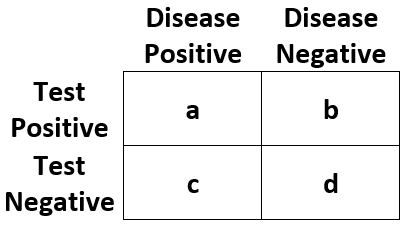test_consequences {dcurves} R Documentation

## Test Consequences

### Description

Test Consequences

### Usage

test_consequences(
formula,
data,
statistics = c("pos_rate", "neg_rate", "test_pos_rate", "test_neg_rate", "tp_rate",
"fp_rate", "fn_rate", "tn_rate", "ppv", "npv", "sens", "spec", "lr_pos", "lr_neg"),
thresholds = seq(0, 1, by = 0.25),
label = NULL,
time = NULL,
prevalence = NULL
)


### Arguments

 formula a formula with the outcome on the LHS and a sum of markers/covariates to test on the RHS data a data frame containing the variables in ⁠formula=⁠. statistics Character vector with statistics to return. See below for details thresholds vector of threshold probabilities between 0 and 1. Default is seq(0, 0.99, by = 0.01). Thresholds at zero are replaced with 10e-10. label named list of variable labels, e.g. list(age = "Age, years") time if outcome is survival, ⁠time=⁠ specifies the time the assessment is made prevalence When NULL, the prevalence is estimated from ⁠data=⁠. If the data passed is a case-control set, the population prevalence may be set with this argument.

### Value

a tibble with test consequences

### statistics

The following diagnostic statistics are available to return.Statistic Abbreviation Definition Outcome Positive Rate "pos_rate" (a + c) / (a + b + c + d) Outcome Negative Rate "neg_rate" (b + d) / (a + b + c + d) Test Positive Rate "test_pos_rate" (a + b) / (a + b + c + d) Test Negative Rate "test_neg_rate" (c + d) / (a + b + c + d) True Positive Rate "tp_rate" a / (a + b + c + d) False Positive Rate "fp_rate" b / (a + b + c + d) False Negative Rate "fn_rate" c / (a + b + c + d) True Negative Rate "tn_rate" d / (a + b + c + d) Positive Predictive Value "ppv" a / (a + b) Negative Predictive Value "npv" d / (c + d) Sensitivity "sens" a / (a + c) Specificity "spec" d / (b + d) Positive Likelihood Ratio "lr_pos" sens / (1 - spec) Negative Likelihood Ratio "lr_neg" (1 - sens) / spec

### Examples

test_consequences(cancer ~ cancerpredmarker, data = df_binary)


[Package dcurves version 0.4.0 Index]